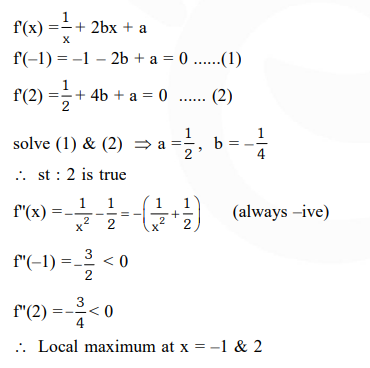Deepak Scored 45->99%ile with Bounce Back Crack Course. You can do it too!

# Solve this following

Question:

Let $a, b \in R$ be such that the function $f$ given by $f(x)=\ln |x|+b x^{2}+a x, x \neq 0$ has extreme values at $\mathrm{x}=-1$ and $\mathrm{x}=2$.

Statement-1 : $\mathrm{f}$ has local maximum at $\mathrm{x}=-1$ and at $\mathrm{x}=2$

Statement-2 : $\mathrm{a}=\frac{1}{2}$ and $\mathrm{b}=\frac{-1}{4}$.

1. Statement-1 is true, Statement $-2$ is false.

2. Statement- 1 is false, Statement- 2 is true.

3. Statement-1 is true, Statement-2 is true ;Statement-2 is a correct explanation for Statement-1.

4. Statement-1 is true, Statement-2 is true ; Statement $-2$ is not a correct explanation for Statement $-1$.

Correct Option: , 3

Solution: